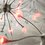# do anybody have reasons for this??

L = 2

L(L-1) = 2(L-1)

L2-L = 2L-2

L2-2L = L-2

L(L-2) = L-2

L = 1

Find the mistake in the above maths equationsNote by Yashasvini Sharma
6 years, 6 months ago

This discussion board is a place to discuss our Daily Challenges and the math and science related to those challenges. Explanations are more than just a solution — they should explain the steps and thinking strategies that you used to obtain the solution. Comments should further the discussion of math and science.

When posting on Brilliant:

• Use the emojis to react to an explanation, whether you're congratulating a job well done , or just really confused .
• Ask specific questions about the challenge or the steps in somebody's explanation. Well-posed questions can add a lot to the discussion, but posting "I don't understand!" doesn't help anyone.
• Try to contribute something new to the discussion, whether it is an extension, generalization or other idea related to the challenge.

MarkdownAppears as
*italics* or _italics_ italics
**bold** or __bold__ bold
- bulleted- list
• bulleted
• list
1. numbered2. list
1. numbered
2. list
Note: you must add a full line of space before and after lists for them to show up correctly
paragraph 1paragraph 2

paragraph 1

paragraph 2

[example link](https://brilliant.org)example link
> This is a quote
This is a quote
    # I indented these lines
# 4 spaces, and now they show
# up as a code block.

print "hello world"
# I indented these lines
# 4 spaces, and now they show
# up as a code block.

print "hello world"
MathAppears as
Remember to wrap math in $$ ... $$ or $ ... $ to ensure proper formatting.
2 \times 3 $2 \times 3$
2^{34} $2^{34}$
a_{i-1} $a_{i-1}$
\frac{2}{3} $\frac{2}{3}$
\sqrt{2} $\sqrt{2}$
\sum_{i=1}^3 $\sum_{i=1}^3$
\sin \theta $\sin \theta$
\boxed{123} $\boxed{123}$

Sort by:

Fifth row, (L-2)=0

- 6 years, 6 months ago

I think the mistake is in the fifth row, where both side divided by (L-2) which is zero

- 6 years, 6 months ago

According to me 5th step is absolutely right but when you do the 2nd step you must mention l is not equal to 1

- 5 years, 4 months ago

Fourth step equates zero to zero

- 6 years, 6 months ago

fourth row.

- 6 years, 6 months ago

or 2

- 6 years, 6 months ago

2L-2 is 0

- 6 years, 6 months ago

No, 2L-2 = 2

- 6 years, 6 months ago

Simple because of multiplying it by (L-1) it become quadratic ..So it has two solutions 1 & 2.

- 6 years, 5 months ago

Fifth St ep is wrongg

- 6 years, 1 month ago